• +91 9971497814
• info@interviewmaterial.com

# RD Chapter 13- Linear Equations in Two Variables Ex-13.4 Interview Questions Answers

### Related Subjects

Question 1 :
Give the geometric representations of the following equations
(a) on the number line (b) on the Cartesian plane:
(i) x = 2 (ii) y + 3 = 0 (iii) y = 3 (iv) 2x + 9 = 0 (v) 3x – 5 = 0

(i) x = 2
The representation of equation on the number line: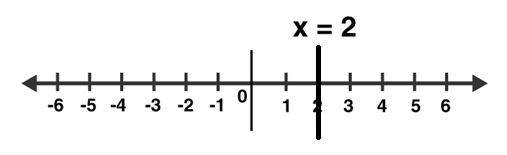The representation of equation on the Cartesian plane: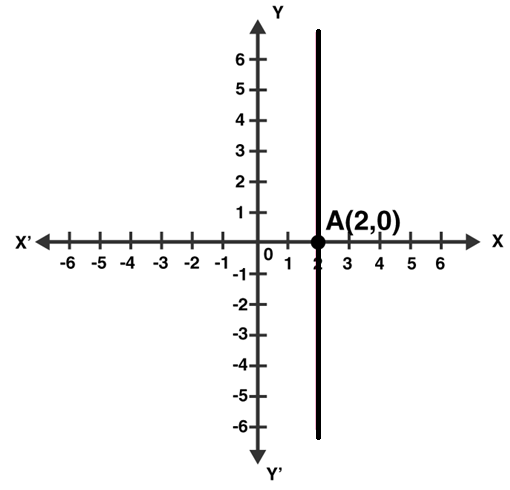(ii) y + 3 = 0
or y = -3
The representation of equation on the number line: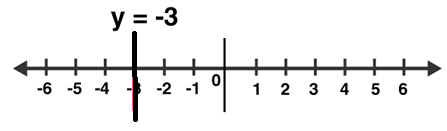The representation of equation on the Cartesian plane: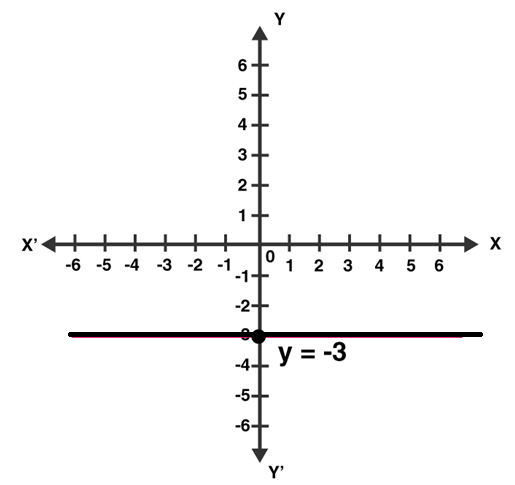(iii) y = 3
The representation of equation on the number line: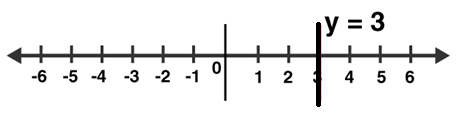The representation of equation on the Cartesian plane: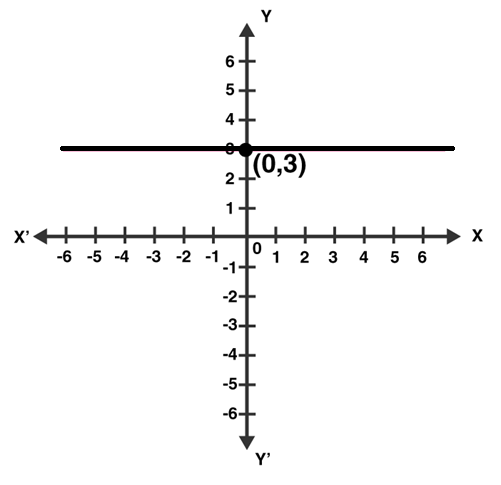(iv) 2x + 9 = 0
or x = -9/2
The representation of equation on the number line: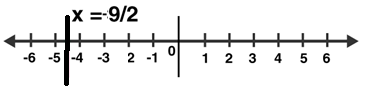The representation of equation on the Cartesian plane: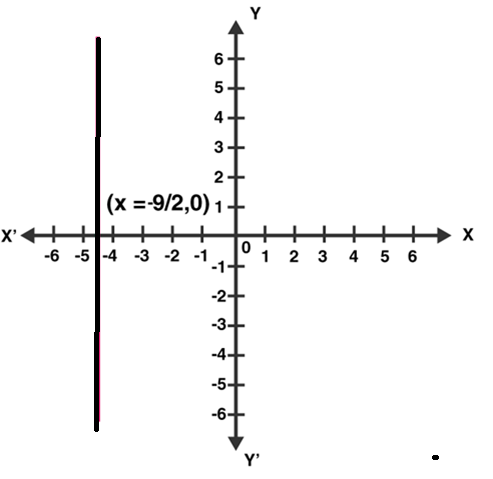(v) 3x – 5 = 0
or x = 5/3
The representation of equation on the number line: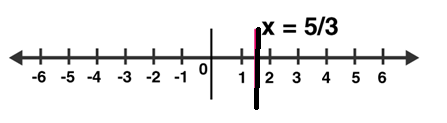The representation of equation on the Cartesian plane: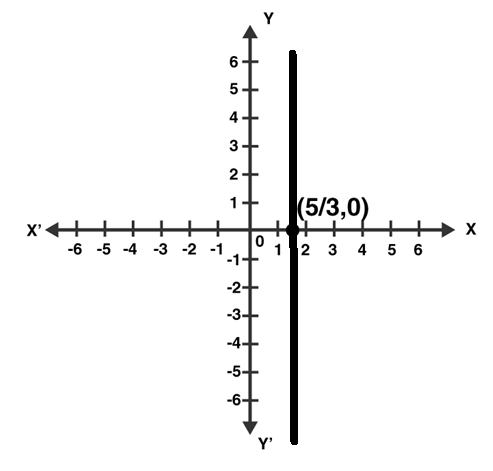Question 2 :
Give the geometrical representation of 2x + 13 = 0 as an equation in
(i) one variable (ii) two variables

2x + 13 = 0
(i) Isolate given equation in x
Subtract 13 from both the sides
2x + 13 – 13 = 0 – 13
2x = -13
Divide each side by 2
x = -13/2 = -6.5
Which is an equation in one variable.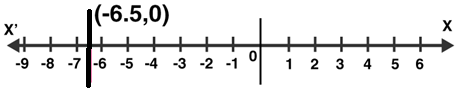(ii) 2x + 13 = 0 can be written as 2x + 0y + 13 = 0
The representation of the solution on the Cartesian plane: A line parallel to y axis passing through the point (-13/2 , 0):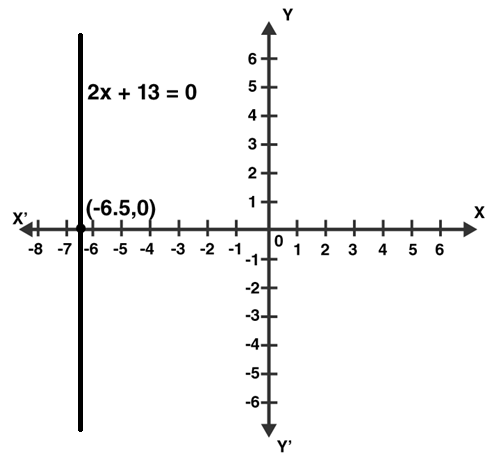Todays Deals### RD Chapter 13- Linear Equations in Two Variables Ex-13.4 Contributorskrishan

Name:
Email:

# Latest News# 9000 interview questions in different categories